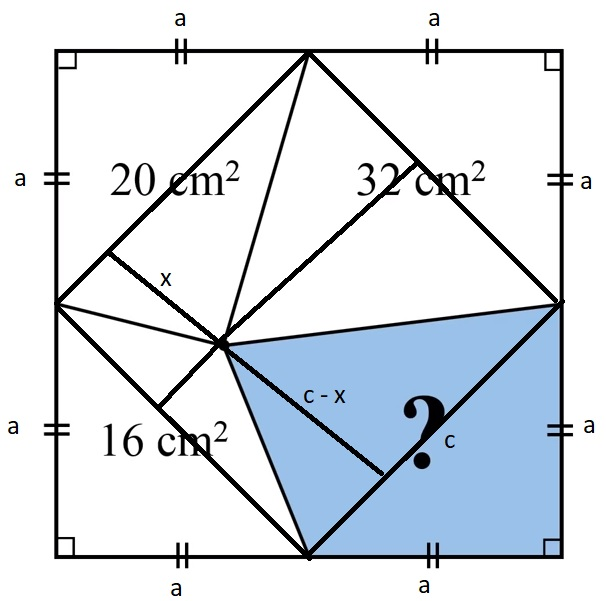Categories

## Another slow solution

Saw this small problem on YouTube, as usual got to the solution but took time + it was not very straight forward like in the video scroll below for it 🙂big total square area = (2a)^2 = 4a^2
corner triangles hipotenuse Pythagorean theorem c^2 = a^2 + a^2 -> c=sqrt(2)a

one corner triangle area = big total square / 8 -> a^2 / 2

20 cm^2 = two triangles area = (a^2 / 2) + (x * sqrt(2)a) / 2
-> a^2 + x * sqrt(2)a – 40 = 0 (1)

blue area n
n = big square area minus 20 minus 32 minus 16
n = 4a^2 – 68
n also two triangles area = (a^2 / 2) + (((sqrt(2)a – x) * sqrt(2)a) / 2
->
2n = 8a^2 – 136 = a^2 + ((sqrt(2)a – x) * sqrt(2)a
7a^2 – 2a^2 + x * sqrt(2)a – 136 = 0
5a^2 + x * sqrt(2)a – 136 = 0 (2)

combining (1) and (2)
5a^2 + x * sqrt(2)a – 136 = a^2 + x * sqrt(2)a – 40

4a^2 = 96
n = 4a^2 – 68
n = 28

Categories

## Pension plan

If a bank or an insurance company asks me if I have any pension plans, the answer will be yes, outline of the plan below:(original found here)

Categories

LG G3 Beat : Product Movie

Categories

## My birthday song #1 – Champaign – How ‘Bout Us

http://geboortedaghit.nl/ddmmyyyy
Interesting concept, you fill in your birthdate, replace ddmmyyyy with your birtdate e.g. http://geboortedaghit.nl/01071978 for July 1st 1978.
What you get as a result is #1 song at that time in charts (not sure which).
This is what I got (never heard it before to be honest). Note the number 22 appear in second 29 🙂
Champaign – How ‘Bout Us

Categories

Categories

## Heartbeat exploit for dummies

xkcd: Heartbleed Explanation

Categories

## Android next version codenames

I have 2 suggestions:

• Apple strudel
• Blackberry pie
Categories

`Size matters not. Look at me.`

Categories

## Thanks all

For the best wishes, it is after all a nice round birthday, 100000.
Hope I make it to 1000000, hell maybe even the next one 😀
Nerd out.

Categories

## Trilogy

Best trilogy since starwars 🙂